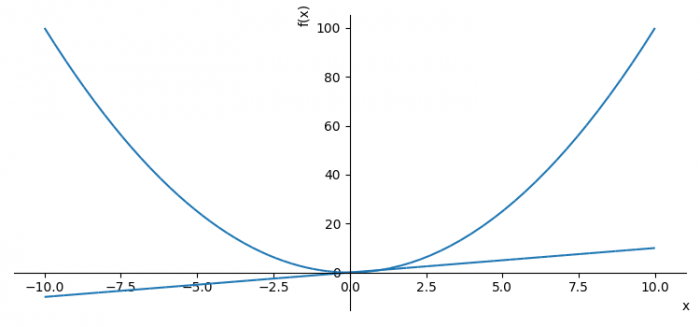# Display two Sympy plots as one Matplotlib plot (add the second plot to the first)

PythonServer Side ProgrammingProgramming

To display two sympy plots as one Matplotlib plot, we can take the following steps −

• Set the figure size and adjust the padding between and around the subplots.
• Transform strings into instances of :class:'Symbol' class.
• Plot a function of a single variable as a curve.
• Use the extend method to add all the series of plot2 (p2) in plot1 (p1).
• To display the figure, use show() method.

## Example

from sympy import symbols
from sympy.plotting import plot
from matplotlib import pyplot as plt

plt.rcParams["figure.figsize"] = [7.50, 3.50]
plt.rcParams["figure.autolayout"] = True

x = symbols('x')

p1 = plot(x*x, show=False)
p2 = plot(x, show=False)
p1.extend(p2)

p1.show()

## Output